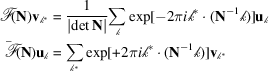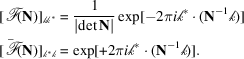International
Tables for
Crystallography
Volume B
Reciprocal space
Edited by U. Shmueli

International Tables for Crystallography (2010). Vol. B, ch. 1.3, p. 51   | 1 | 2 |

## Section 1.3.2.7.4. Matrix representation of the discrete Fourier transform (DFT)

G. Bricognea

aGlobal Phasing Ltd, Sheraton House, Suites 14–16, Castle Park, Cambridge CB3 0AX, England, and LURE, Bâtiment 209D, Université Paris-Sud, 91405 Orsay, France

#### 1.3.2.7.4. Matrix representation of the discrete Fourier transform (DFT)

| top | pdf |

By virtue of definitions (i) and (ii),so thatandmay be represented, in the canonical bases ofand, by the following matrices:When N is symmetric,andmay be identified in a natural manner, and the above matrices are symmetric.

When N is diagonal, say, then the tensor product structure of the full multidimensional Fourier transform (Section 1.3.2.4.2.4)gives rise to a tensor product structure for the DFT matrices. The tensor product of matrices is defined as follows:Let the index vectorsandbe ordered in the same way as the elements in a Fortran array, e.g. forwithincreasing fastest,next fastest,slowest; thenwhereandwhere